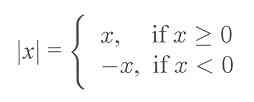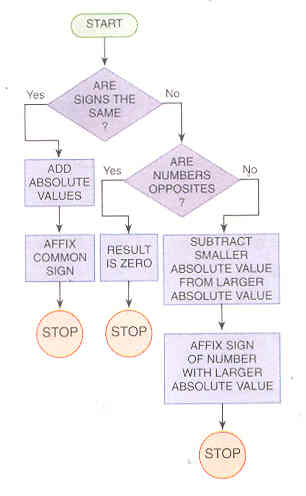# Section 5.3: Integers

## 5.3 Outline

1. Introduction
1. zero
2. whole numbers
3. opposites
4. integers
5. absolute value
1. procedure
2. flowchart
3. Multiplication of integers
1. procedure
2. flowchart
4. Subtraction of integers
5. Division of integers
1. definition
2. procedure
3. NEVER DIVIDE BY ZERO

## 5.3 Essential Ideas

This section, which may be review, is concerned with the integers and operations with integers. The first essential idea of this section is the notion of absolute value.
The absolute value of x, denoted by |x| is defined asThe operations of addition, subtraction, multiplication, and division or integers were defined: